# SPICE distortion anomaly

#### Ian0

Joined Aug 7, 2020
6,980
Of the two plots, the stepped one has R1 and R2 stepped from 10 ohms to 100 ohms, 6 steps per decade, the other has R1 and R2 fixed at 33 ohms.
Any ideas why the 3rd harmonic distortion is an order of magnitude better in the fixed value plot?
Am I correct in using {R} for both values for the stepped plot? and the command is ".step dec param R 10 100 6"? Or am I doing something wrong?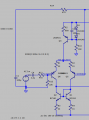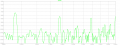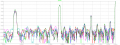#### eetech00

Joined Jun 8, 2013
3,418
Of the two plots, the stepped one has R1 and R2 stepped from 10 ohms to 100 ohms, 6 steps per decade, the other has R1 and R2 fixed at 33 ohms.
Any ideas why the 3rd harmonic distortion is an order of magnitude better in the fixed value plot?
Am I correct in using {R} for both values for the stepped plot? and the command is ".step dec param R 10 100 6"? Or am I doing something wrong?
View attachment 228414View attachment 228415View attachment 228416
Step the value of R from 10 to 100 in steps of 6
10 16 22 28 34.....100

Wait...dec

Values it will use fro R:
.step r=10
.step r=14.678
.step r=21.5443
.step r=31.6228
.step r=46.4159
.step r=68.1292
.step r=100

You can see this in the log file.

Last edited:

#### Ian0

Joined Aug 7, 2020
6,980
Step the value of R from 10 to 100 in steps of 6
10 16 22 28 34.....100

Wait...dec

Values it will use fro R:
.step r=10
.step r=14.678
.step r=21.5443
.step r=31.6228
.step r=46.4159
.step r=68.1292
.step r=100

You can see this in the log file.
The fixed value in the other plot is 33 ohms, so I would not expect the distortion at 33 ohms to be significantly different to the distortion at 31.6228 ohms, especially as all 7 values in the stepped plot give the same figure. That's what puzzled me.
(6 steps per decade correspond approximately to every E6 resistor value)

#### Ian0

Joined Aug 7, 2020
6,980
I also get ten times worse distortion whenever the step command is used, even if no value is being stepped.

#### ericgibbs

Joined Jan 29, 2010
17,136
hi Ian,
What I would do to check out that LTSpice is giving misleading results, is to create a simple test circuit and subject it to the same .step commands etc ..

If you create a simple version of your circuit, please post the asc file and we can compare results.
E

#### eetech00

Joined Jun 8, 2013
3,418
The fixed value in the other plot is 33 ohms, so I would not expect the distortion at 33 ohms to be significantly different to the distortion at 31.6228 ohms, especially as all 7 values in the stepped plot give the same figure. That's what puzzled me.
(6 steps per decade correspond approximately to every E6 resistor value)
If you want to step thru all E6 values, here's an example. It doesn't round but should be close enough.

I've attached an example file.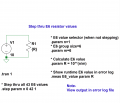#### Attachments

• 721 bytes Views: 2
•ericgibbs

#### Ian0

Joined Aug 7, 2020
6,980
hi Ian,
What I would do to check out that LTSpice is giving misleading results, is to create a simple test circuit and subject it to the same .step commands etc ..

If you create a simple version of your circuit, please post the asc file and we can compare results.
E
I don't know if I can make a version using only components that come with the LTSpice installation.
Here's the circuit.
I wondered if {R} was not sufficiently unique, so I changed it to {DEGEN} but it made no difference.
With no step command (R1=R2=33 ohm) 3rd harmonics is -66dB.
With R1 and R2 stepped from 10 ohms to 100 ohms, 3rd harmonic is -56dB for all 7 steps
With the step command still active, but no resistor called {DEGEN} the 3rd harmonics is -56dB

#### Attachments

• 6.2 KB Views: 7

#### eetech00

Joined Jun 8, 2013
3,418
I don't know if I can make a version using only components that come with the LTSpice installation.
Here's the circuit.
I wondered if {R} was not sufficiently unique, so I changed it to {DEGEN} but it made no difference.
With no step command (R1=R2=33 ohm) 3rd harmonics is -66dB.
With R1 and R2 stepped from 10 ohms to 100 ohms, 3rd harmonic is -56dB for all 7 steps
With the step command still active, but no resistor called {DEGEN} the 3rd harmonics is -56dB
I corrected some model/symbols (included).

Simply unzip, browse to the unzipped "njdquasicomp-1" folder, and run the .asc file (no need to move files around).

#### Attachments

• 6.8 KB Views: 3

#### Ian0

Joined Aug 7, 2020
6,980
I corrected some model/symbols (included).

Simply unzip, browse to the unzipped "njdquasicomp-1" folder, and run the .asc file (no need to move files around).
Many thanks for the proper BCV61, but the original problem still remains.
With R1 and R2 fixed at 33 ohms, the distortion is -74dB and -65dB for 2nd and 3rd harmonics
With R1 and R2 stepped from 10 ohms to 100 ohms, the distortion for the 33 ohm step is -62dB and -57dB

#### eetech00

Joined Jun 8, 2013
3,418
Many thanks for the proper BCV61, but the original problem still remains.
With R1 and R2 fixed at 33 ohms, the distortion is -74dB and -65dB for 2nd and 3rd harmonics
With R1 and R2 stepped from 10 ohms to 100 ohms, the distortion for the 33 ohm step is -62dB and -57dB
I'm assuming you want to keep the input balanced so I'm stepping both R1 and R2 and the same time from 10 to 100 ohms.
(The result is the same if I used a fixed value of 33 ohms or a stepped value of 33 ohms)
See below.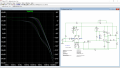Last edited:

#### eetech00

Joined Jun 8, 2013
3,418
Also, It will help if you labeled the wire where you are making your measurement so we all measure at the same point.
Otherwise, the net name can change occasionally.

#### Ian0

Joined Aug 7, 2020
6,980
Also, It will help if you labeled the wire where you are making your measurement so we all measure at the same point.
Otherwise, the net name can change occasionally.
I wondered how to stop that - it's very irksome when it changes the net name when I don't realise it has happened!
The output is at the junction of L1 and R11.

#### Ian0

Joined Aug 7, 2020
6,980
I'm assuming you want to keep the input balanced so I'm stepping both R1 and R2 and the same time from 10 to 100 ohms.
(The result is the same if I used a fixed value of 33 ohms or a stepped value of 33 ohms)
See below.
Correct - R1 and R2 are always the same value.
The output is from the FFT (Blackman window) from a transient analysis ".tran 0 100m 0 100n" with a 300mV peak 1kHz sinewave on the input, taking the value of the peaks at 2kHz and 3kHz as the 2nd and 3rd harmonic distortion.

#### eetech00

Joined Jun 8, 2013
3,418
Correct - R1 and R2 are always the same value.
The output is from the FFT (Blackman window) from a transient analysis ".tran 0 100m 0 100n" with a 300mV peak 1kHz sinewave on the input, taking the value of the peaks at 2kHz and 3kHz as the 2nd and 3rd harmonic distortion.
Hmmm...i see what you mean.

I got two different FFT readings using 31.6228 for R1 and R2.
But they should be the same...

I ran one sim using .param n=9 while commenting out the ".step" statement.
Then
I ran two sims with the step statement set to: ".step param n list 9 10" (it won't let you list one step)

I must be overlooking something..

#### Ian0

Joined Aug 7, 2020
6,980
Hmmm...i see what you mean.

I got two different FFT readings using 31.6228 for R1 and R2.
But they should be the same...
Perhaps I'm expecting too much from it? Should it really be able to predict distortion to that level of accuracy? Perhaps it's something to do with its generation of thermal noise, which is only a few dB below the level at which we are working.

#### eetech00

Joined Jun 8, 2013
3,418
Perhaps I'm expecting too much from it? Should it really be able to predict distortion to that level of accuracy? Perhaps it's something to do with its generation of thermal noise, which is only a few dB below the level at which we are working.
But I would expect them to still be the same because we are not changing any conditions.

#### eetech00

Joined Jun 8, 2013
3,418
Perhaps I'm expecting too much from it? Should it really be able to predict distortion to that level of accuracy? Perhaps it's something to do with its generation of thermal noise, which is only a few dB below the level at which we are working.
OK.

Always add this statement (as a directive) to the schematic before you run the simulation:

.opt plotwinsize=0

This turns off waveform compression and prevents missed data points.

Last edited:

#### Ian0

Joined Aug 7, 2020
6,980
I was expecting it to make it run more slowly, but it actually seems quicker.
Now I have an amplifier that claims distortion of -90dBV for a 10V output. The thermal noise contribution from the lower feedback resistor is about -90dBV. My pcb layout skills will need to be on top form if I can achieve that in real life! Unfortunately, I won't know until the end of lockdown (when I can go and see my colleague who has an AP Audio Analyser) what I have achieved.

#### eetech00

Joined Jun 8, 2013
3,418
Good luck with your project.